#### Runge-Kutta methods

Although Euler integration is efficient and easy to understand, it generally yields poor approximations. Taking a Taylor series expansion of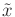at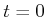yields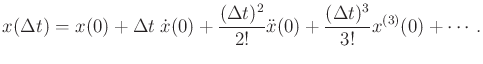(14.17)

Comparing to (14.16), it can be seen that the Euler method just uses the first term of the Taylor series, which is an exact representation (ifis analytic). Thus, the neglected terms reflect the approximation error. If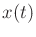is roughly linear, then the error may be small; however, if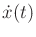or higher order derivatives change quickly, then poor approximations are obtained.

Runge-Kutta methods are based on using higher order terms of the Taylor series expansion. One of the most widely used and efficient numerical integration methods is the fourth-order Runge-Kutta method. It is simple to implement and yields good numerical behavior in most applications. Also, it is generally recommended over Euler integration. The technique can be derived by performing a Taylor series expansion at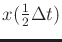. This state itself is estimated in the approximation process.

The fourth-order Runge-Kutta integration method is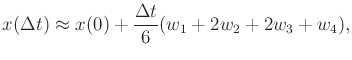(14.18)

in which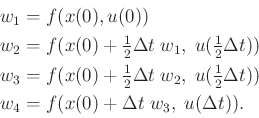(14.19)

Although this is more expensive than Euler integration, the improved accuracy is usually worthwhile in practice. Note that the action is needed at three different times: 0,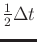, and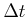. If the action is constant over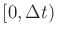, then the same value is used at all three times.

The approximation error depends on how quickly higher order derivatives ofvary over time. This can be expressed using the remaining terms of the Taylor series. In practice, it may be advantageous to adaptover successive iterations of Runge-Kutta integration. In , for example, it is suggested thatis scaled by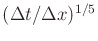, in which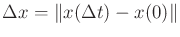, the Euclidean distance in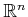.

Steven M LaValle 2020-08-14